NEET  >  Test: Electric Charges & Fields

# Test: Electric Charges & Fields

Test Description

## 20 Questions MCQ Test | Test: Electric Charges & Fields

Test: Electric Charges & Fields for NEET 2023 is part of NEET preparation. The Test: Electric Charges & Fields questions and answers have been prepared according to the NEET exam syllabus.The Test: Electric Charges & Fields MCQs are made for NEET 2023 Exam. Find important definitions, questions, notes, meanings, examples, exercises, MCQs and online tests for Test: Electric Charges & Fields below.
Solutions of Test: Electric Charges & Fields questions in English are available as part of our course for NEET & Test: Electric Charges & Fields solutions in Hindi for NEET course. Download more important topics, notes, lectures and mock test series for NEET Exam by signing up for free. Attempt Test: Electric Charges & Fields | 20 questions in 30 minutes | Mock test for NEET preparation | Free important questions MCQ to study for NEET Exam | Download free PDF with solutions
 1 Crore+ students have signed up on EduRev. Have you?
Test: Electric Charges & Fields - Question 1

### Which of the following methods can be used to charge a metal sphere positively without touching it . Select the best

Detailed Solution for Test: Electric Charges & Fields - Question 1

This can be possible by induction, if a negative charge rod is placed near the sphere (at a distance not in touch) than it attracts positive charge inside the sphere towards its side and the negative charges collected on other side and when the surface of sphere connected to earth than the negative charge goes to earth and the sphere remain positively charged.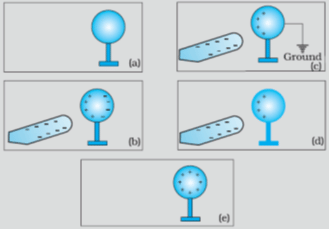Test: Electric Charges & Fields - Question 2

### When a negatively charged conductor is connected to earth,

Detailed Solution for Test: Electric Charges & Fields - Question 2

After earthing a positively charged conductor electrons flow from earth to conductor and if a negatively charged conductor is earthed then electrons flows from conductor to earth.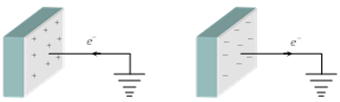Test: Electric Charges & Fields - Question 3

### A point charge of 2.0 μC is at the centre of a cubic Gaussian surface 9.0 cm on edge. What is the net electric flux through the surface?

Detailed Solution for Test: Electric Charges & Fields - Question 3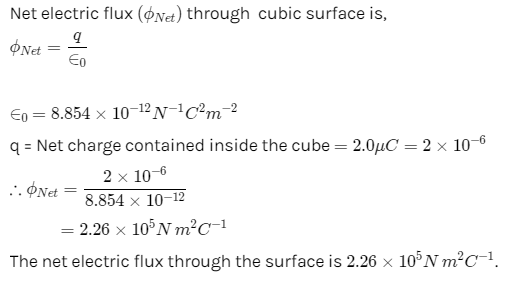Test: Electric Charges & Fields - Question 4

Two identical conductors of copper and aluminium are placed in an identical electric field. The magnitude of induced charge in the aluminium will be:

Detailed Solution for Test: Electric Charges & Fields - Question 4

As aluminium and copper are metals, their mobile electrons move under the influence of the external field until they reach the surface of the metal and collect there. External electric fields induce surface changes on metal objects that exactly cancel the field within. Since the field applied is same in both case, the induced charge will be the same. Hence, the magnitude of induced charge in the aluminium will be the same as that of copper.

Test: Electric Charges & Fields - Question 5

A point charge causes an electric flux of −1.0×103Nm2/C to pass through a spherical Gaussian surface of 10.0 cm radius centred on the charge.
(a) If the radius of the Gaussian surface were doubled, how much flux would pass through the surface?
(b) What is the value of the point charge?

Detailed Solution for Test: Electric Charges & Fields - Question 5

Electric flux, Φ = −1.0 × 103 N m2/C

r = 10.0 cm

Electric flux piercing out through a surface depends on the net charge enclosed inside a body. It does not depend on the size of the body. If the radius of the Gaussian surface is doubled, then the flux passing through the surface remains the same i.e., −103 N m2/C.

Electric flux is given by the relation,

qϕ=q∈0

Where,

q = Net charge enclosed by the spherical surface

0 = Permittivity of free space = 8.854 × 10−12 N−1 Cm−2

∴ qq=ϕ∈0

= −1.0 × 103 × 8.854 × 10−12

= −8.854 × 10−9 C

= −8.854 nC

Therefore, the value of the point charge is −8.854 nC.

Test: Electric Charges & Fields - Question 6

Electric charge:

Detailed Solution for Test: Electric Charges & Fields - Question 6

Electric charge, the basic property of matter carried by some elementary particles, such as atoms; ions; electrons; etc, that govern how the particles are affected by an electric or magnetic field.
Electric charge, which can be positive or negative, occurs in discrete natural units and is neither created nor destroyed.

Test: Electric Charges & Fields - Question 7

An electric dipole is

Detailed Solution for Test: Electric Charges & Fields - Question 7

An electric dipole is a separation of positive and negative charges. The simplest example of this is a pair of electric charges of equal magnitude but opposite sign, separated by some (usually small) distance. A permanent electric dipole is called an electret.

Test: Electric Charges & Fields - Question 8

A hollow spherical conductor of radius 2m carries a charge of 500 μ C. Then electric field strength at its surface is

Detailed Solution for Test: Electric Charges & Fields - Question 8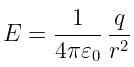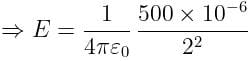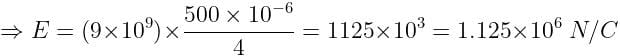Test: Electric Charges & Fields - Question 9

For forming an electric dipole between two point charges separated by a small distance, the two point charges are of

Detailed Solution for Test: Electric Charges & Fields - Question 9

An electric dipole is tagged as a pair of objects which possess equal & opposite charges, parted by a significantly small distance.

Test: Electric Charges & Fields - Question 10

The amount of work done in moving a charge from one point to another along an equipotential line or surface charge is

Detailed Solution for Test: Electric Charges & Fields - Question 10

Since Potential difference between two points in equipotential surfaces is zero , the work done between two points in equipotential surface is also zero .

Test: Electric Charges & Fields - Question 11

An electric field can deflect

Detailed Solution for Test: Electric Charges & Fields - Question 11

Only alpha rays are moving with small velocity and having charge so they will be affected by electric field.

Test: Electric Charges & Fields - Question 12

Under the influence of the coulomb field of charge +Q, a charge −q is moving around it in an elliptical orbit. Find out the correct statement(s).

Detailed Solution for Test: Electric Charges & Fields - Question 12

Since the charge –q is moving in elliptical orbit so to make its motion stable the total angular momentum of the charge is constant since it experience a centripetal force from the charge +Q so it follow the motion as the motion of earth around sun.

Test: Electric Charges & Fields - Question 13

Conservation of charges in tribo electric charging

Detailed Solution for Test: Electric Charges & Fields - Question 13

The triboelectric effect (also known as triboelectric charging) is a type of contact electrification in which certain materials become electrically charged after they come into frictional contact with a different material.
Example: Rubbing glass with fur, or a plastic comb through the hair.

When we charge something with static electricity, no electrons are made or destroyed. Electrons are just moved from one place to another. The net electric charge stays the same. This is called the principle of conservation of charge.
i.e. If a glass rod is rubbed with silk, some electrons moves from glass rod to silk no new charge is generated or destroyed.

Test: Electric Charges & Fields - Question 14

Consider a neutral conducting sphere. A positive point charge is placed outside the sphere. The net charge on the sphere is then,

Detailed Solution for Test: Electric Charges & Fields - Question 14

If a charge q is placed outside than the electric field lines incident on the conducting sphere , if some charge is developed due to thee lines than opposite surface becomes oppositely charge and the total charge becomes zero.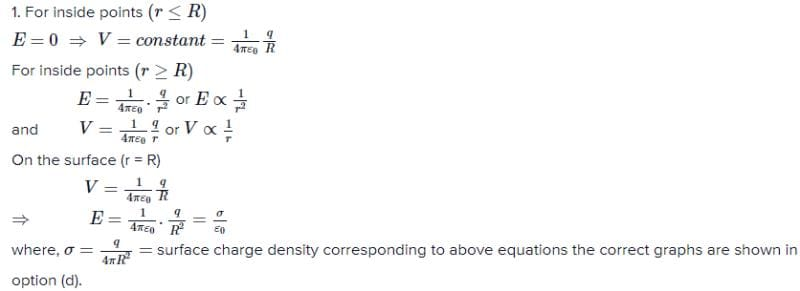Test: Electric Charges & Fields - Question 15

Two positive charges

Detailed Solution for Test: Electric Charges & Fields - Question 15

Acc to coulomb's law unlike charges always attract each other while like charges always repel each other.

Test: Electric Charges & Fields - Question 16

Ionization of a neutral atom is the

Detailed Solution for Test: Electric Charges & Fields - Question 16

It is not possible to remove or add protons/neutrons to an atom, but electrons can be added or removed by an atom easily.
By adding electrons it becomes negatively charged.
By removing electrons it becomes positively charged.

Test: Electric Charges & Fields - Question 17

A charge q is placed at the center of the line joining two equal charges Q. The system of the three charges will be in equilibrium if q is equal to

Detailed Solution for Test: Electric Charges & Fields - Question 17

The situation is as shown in the figure.Let two equal charges Q each placed at points A and B at a distance r apart. C is the centre of AB where charge q is placed.

For equilibrium, net force on charge Q= 0Test: Electric Charges & Fields - Question 18

The electrostatic force is:

Detailed Solution for Test: Electric Charges & Fields - Question 18

The electrostatic force is an attractive and repulsive force between particles are caused due to their electric charges. The electric force between stationary charged bodies is conventionally known as the electrostatic force.

Test: Electric Charges & Fields - Question 19

Two charges A = -2.50 μC  and B = 6.0 μC are at a distance of 1 meter from each other. Distance from A at which the electric field is zero in meters is

Detailed Solution for Test: Electric Charges & Fields - Question 19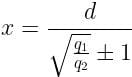where,
x = distance of the null point from the small charge
d = distance between two charges
q= bigger charge
q= small charge
+1 for like charges and -1 for unlike charges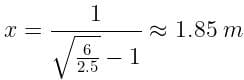Test: Electric Charges & Fields - Question 20

Conductors are materials

Detailed Solution for Test: Electric Charges & Fields - Question 20

Conductors are materials that permit electrons to flow freely from particle to particle. An object made of a conducting material will permit a charge to be transferred across the entire surface of the object.

 Use Code STAYHOME200 and get INR 200 additional OFF Use Coupon Code
Information about Test: Electric Charges & Fields Page
In this test you can find the Exam questions for Test: Electric Charges & Fields solved & explained in the simplest way possible. Besides giving Questions and answers for Test: Electric Charges & Fields, EduRev gives you an ample number of Online tests for practice

### How to Prepare for NEET

Read our guide to prepare for NEET which is created by Toppers & the best Teachers